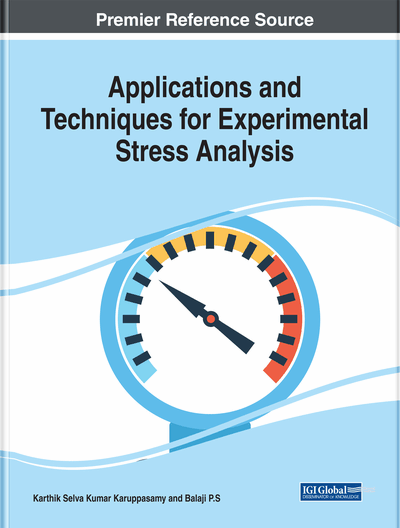# Introduction to Stress-Strain Relationship and Its Measurement Techniques

Karthik Selva Kumar Karuppasamy, Balaji P. S., Niranjan Sahoo
DOI: 10.4018/978-1-7998-1690-4.ch002
OnDemand:
(Individual Chapters)
Available
\$29.50
No Current Special Offers

## Abstract

In this chapter, the basic concepts of strain and the subject of components deformation and associated strain is proposed to be briefly discussed. The strain is a pure geometric quantity so there will be no limitation on the material of the component is required. The significant aspects related to strain and stress-strain relationship briefly discussed in this proposed chapter were as follows: displacement and strain, principal strains, compatibility equations, relationship between stress and strain, two and three dimensional state of stress with respect to strain, stress-strain relationship curve, and overview of various experimental techniques employed for the measurement of stress and strain respectively.
Chapter Preview
Top

## Introduction

The components that researchers employ in various engineering applications are exposed to numerous loading conditions, which induces stress and component failures. In the same way, the structural components placed in the flow path of fluids with high currents leads to distortion because of the high stress and strain concentration on the material surfaces, which further leads to the catastrophe of the engineering components (Karthik & Dhas, 2015, 2016, 2018). The failures from stress can be caused by excessive vibrations (Balaji et al., 2015; Leblouba et al., 2015; Balaji et al., 2016a; 2016b). Moreover, thermal stresses can be caused from the temperature distribution on the structural component (Balaji & Yadava, 2013). In the previous chapter, the authors discussed that the states of stress developed at a particular point or location in a body due to the force or load acting on the surface of the structure. To estimate the nature of stress-strain on the materials, it is important to know the graphical measure of mechanical properties of various materials. All the design engineers, university students, and academicians, who are all study or analyze the mechanics of materials, have to understand the relationship between stress and strain. This chapter mostly discusses stress and strain and their relationship. The movement of the arbitrary point due to the applied forces is a vector quantity known as displacement. In addition, every single point in a structure undergoes displacement due to applied forces that have a unique signature on displacement vectors. Each displacement component is matched with respect to the Cartesian coordinates in x, y, and z directions. Researchers can represent the motions of the structure by considering the rotational movement and translational movements as a single component. Subsequently, the authors considered the movement of the points in the structures to be relative to one another. In this chapter, the authors present a detailed overview of the numerous experimental techniques employed to measure the stress and strain.

### Engineering Stress

Engineering stress is the ratio between the load applied to the original cross-sectional area of the material. Also, researchers consider engineering stress as nominal stress.

### True Stress

True stress is the ratio between the load applied to the actual cross-sectional area of the deformed material.

### True Strain

Researchers refer to true strain as the natural log of the quotient of deformed length after loading over the original length before loading.

### Engineering Strain

Researchers refer to engineering strain as the quantity of material deformation per unit length. This is also referred as the nominal strain.

### Lateral Strain

In continuum mechanics, researchers consider the mechanical behavior of the modeled materials to be a single mass instead of distinct masses. Due to this consideration, the distortion of material in the longitudinal direction causes a transverse strain, also called a lateral strain. Lateral strain is the ratio between the variation in lateral dimension to the original lateral dimension.

### Shear Strain

Shear strain is the ratio of the variation in distortion to its actual dimension (length) at right angles to the axes of the subjected component under shear load.

Top

## Two Dimensional Aspects Of Strain

The two-dimensional aspects of the strain are more or less identical to the understanding of strain in the one-dimensional aspects, in which the authors defined the deformation of material by visualizing the material considered to be the group of line elements in lesser amount (Boresi & Chong, 2000). When a test specimen starts to deform, resulting in the development of stretching on the line elements, alternatively, it starts to diminish to revolve around in the space relative to one another. Thus, researchers comprehend the displacement of the line elements with respect to the indication of strain. The strain at a particular point is simply the outcome of the stretching of the line element, due to the contraction and the rotation of the whole elements originating from that particular position. Figure 1 illustrates the occurrence of strain in the z- and y-axis, respectively.

## Complete Chapter List

Search this Book:
Reset